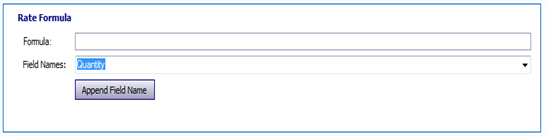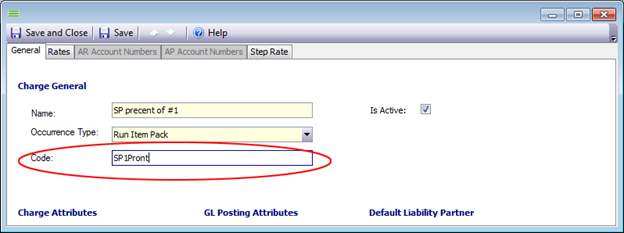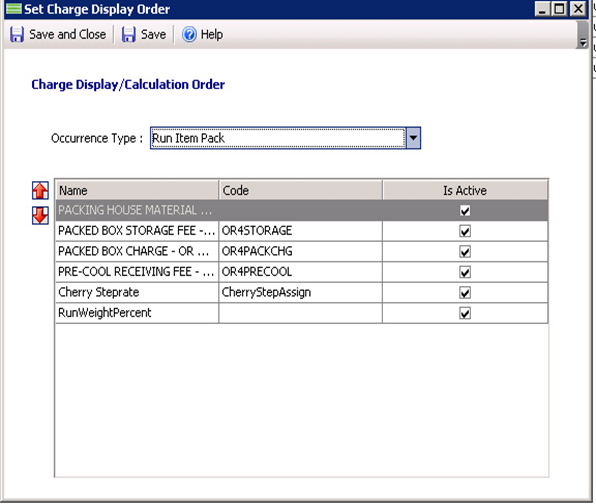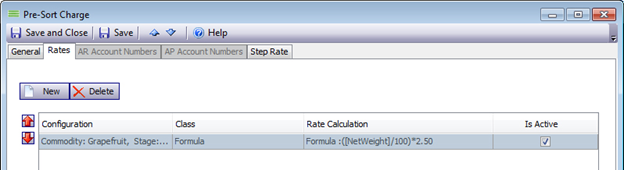# Understanding Charge Formulas

2017-05-18 00:58

Required Vocabulary

Algebraic Expression - In mathematics, an algebraic expression is an expression built up from constantsvariables, and a finite number of Arithmetic operations (additionsubtractionmultiplicationdivision and exponentiation)

Arithmetic Operators -

•  -  Subtraction
•  *  Multiplication
•  /  Division

*, / will be performed before + or - in any expression. Brackets can be used to force a different order of evaluation.

Charge - An expense or cost

Formula - A standard procedure for solving a class of mathematical problems

Numeric Constants - one or more digits, 0 through 9

Prorate - To divide, distribute, or assess proportionately.

Understanding Formulas

• In EnvioAg, Formulas are found in two places: the Settlement, Average, & Final Price Calculations and as a basic Charge Class (Way to calculate a charge).
• A formula enables you to create an algebraic expression that can combine EnvioAg fields, arithmetic operators, and numeric constants together to get a numerical result. In layman's terms, A formula allows you to make mathematical solution based on numbers generated in Envio in order to get a number.
• Formulas are useful when creating prorated amounts.
• The formula set up screen in EnvioAg looks similar to the screen capture shown below. When first opening the window, the formula field will be blank. The Field Names dropdown offers a list of EnvioAg Data fields that can be used to make a calculation: See Anatomy of a Charge for a more in depth explanation of Creating Charges:
•• The choices at the top of the list in the Field Names dropdown correspond to the Charge's OccurrenceType. Example: If you choose a RunItemPack Charge, the top listed field names will be from the Run and Items associated with Packout. If the formula is for a PO Received Item, the fields shown first will be Purchase Order and Item related fields.
•  Any fields used in a formula must be wrapped in square brackets. Examples: [MyField] or [Run.PackedQuantity].
• Parenthesis may be used for higher level math formulas. Rules of Parenthesis follow standard Algebraic rules of notation. If the desired result is the sum of A+B divided by C, the formula would be written: (A+B) / C
1. The Field Name list of charges is shown as a single entity (top choices) up to a very long string of business object options separated by periods. Combinations of business objects may be strung together into long sections.To create the formula, a code is assigned on the General Tab of the Charge window.

• The code must be alphanumeric (no symbols, punctuation, or special characters) because it can be used in other formulas. Example: SP1Pront - This code will be the formula's business object.
• Any charges created on the Item level (when the Occurrence Type choice includes the word Item) are made available in the field list and can be selected and used in other formulas. Example: When looking for the SP1Pront code in the Formula list, it would be on the end of the business object string. Example: Run.ChargeCode.SP1Pront.
• Try to avoid using a Code business object in a formula from the same occurrence type. Charges within the same Occurrence Type are not processed in a specific order (unless you go to configuration and set the specific charge order) and the results could be unpredictable. Avoid scenarios like this: (Run.ChargeCode.SP1Pront.* Run.ChargeAmount.RDTWO) if possible.
• To order the way charges are brought in, Right click on the Charge grid heading row and select Set Charge Display Order. A window  will appear and you can choose the type of charge and arrange from top to bottom which charges will run first.
•Formulas are available in the Following Occurrence types:
• Purchase Order Direct Ship Item Receive
• Run
• Run Item Dump
• Run Item Pack
• Settlement Item Final Price Charge
• Settlement Item Settle Price Charge

Writing  & Understanding Formula formats.

When creating formulas and when using braces, the number of ( facing braces must equal the number of ) facing braces.
The braces tell the software to put everything between them together and, just as in math, commands it to "Do this first".

Example:  A - B * C  may compute differently than (A - B) * C  or  A - (B * C)

In real numbers:  7 - 4 * 5 = 15  ,      (7 - 4) * 5 = 15           or               7 - (4 * 5) = -13

Example in EnvioAg formula logic  ([NetWeight] / 100) * 2.50

Braces may change the result of the equation

In a formula using more than one set of braces, the math is computed from the inner most bracket set, outward using the concept of PEMDAS -- calculate Parenthesis, then Exponents, Multiplication, Division, Addition and Subtraction in that order. (BEDMAS in Canada; B for Brackets)

Click for more thorough information on the Order of Operations and PEMDAS

Consider the following example of a Charge in EnvioAg

(([Run.ChargeAmount.RCOLDChg]/ [Run.PackedNetWeight])* [NetWeight])

Information inside the Red braces will be calculated first, and then the information inside the Blue braces will be calculated next.. (Remember that each set of words in the [ ] braces will be "filled" with a piece of numerical data.)

EnvioAg does all the math, so you never need to do the following, but for a clearer understanding follow along with the math below. If this charge was converted to the numbers that the titles represented...
The information within the  Red braces would be      ((\$2,500  /  10,000)* [NetWeight])  which would be   ((0.25) * [NetWeight]) and once a single number is within a set of braces, the braces can be dropped (0.25* [NetWeight])
Then the information within the Blue braces will be computed. (0.25* 8,540)  and the final answer is computed.

Other Examples:  ([NetWeight]/100)*2.50(( [Run.ChargeAmount.Dump] /  [Run.PackedQuantity]) *  [Quantity])

( [Item.ChargeAmount.TIC])

( RawReceipt.DumpedQuantity] * 9.00)

((([TotalNetAmount]-( [TotalNetAmount]* [Product.SalesOrderProduct.ChargeRate.CommRate]))/ [TotalQuantity]) - [TotalCostAmount])